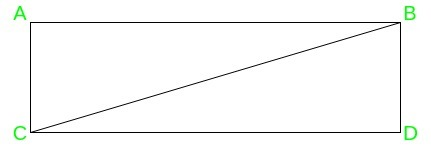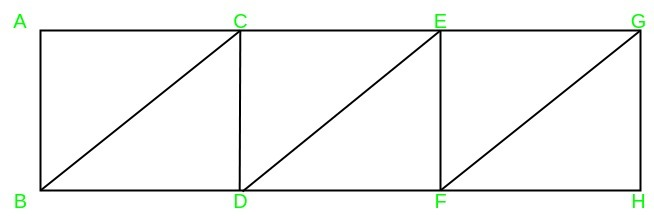# Dividing the rectangle into n right-angled triangles

Puzzle: Find all values of n > 1 for which one can divide a rectangle into n right-angled triangles.

We can divide any rectangle into n right-angled triangles, for any n > 1.

There are two methods to divide:

• Method 1:
1. Case 1: n = 2, we can get two right-angled triangles by cutting the rectangle along the diagonal as shown in the figure.

For example: In the rectangle ABDC, we draw a diagonal BC and get two right-angled triangles, BDC and ABC.2. Case 2: n > 2, we first cut the rectangle along the diagonal and continue with n-2 cuts of any of the available right-angled triangles into two right-angled triangles, by cutting them along the height onto its hypotenuse.

For example: Consider the case of n = 6, in the rectangle ABDC, we first draw diagonal BC, and then (n-2) i.e 4 cuts are made in the triangle ABC along with the height onto the hypotenuse, to get six right-angled triangles. These are BDC, ACE, AEF, FEG, FGH, HGB.

• Method 2: We can also solve the puzzle by considering it case-wise.
1. Case 1: n is even: divide the rectangle into n/2 small rectangles and then can divide each of the smaller rectangle obtained, along its diagonal.

For example: For n=6, in the rectangle AGHB, we first form 3 smaller rectangles as ABCD, CEFD, EGHF. Then, we divide each rectangle along its hypotenuse BC, DE, FG respectively, to get 6 right-angled triangles2. Case 2: n is odd: In this case, first, divide the rectangle into n-1 small triangles using the approach mentioned above and then can cut any of the triangles along with the height onto its hypotenuse.

For example: For n=7, we first form 6 triangles as mentioned in the above point, and then we cut triangle ABC along with the height onto its hypotenuse, to obtain the 7th triangle AIB.

Attention reader! Don’t stop learning now. Get hold of all the important DSA concepts with the DSA Self Paced Course at a student-friendly price and become industry ready.

My Personal Notes arrow_drop_upCheck out this Author's contributed articles.

If you like GeeksforGeeks and would like to contribute, you can also write an article using contribute.geeksforgeeks.org or mail your article to contribute@geeksforgeeks.org. See your article appearing on the GeeksforGeeks main page and help other Geeks.

Please Improve this article if you find anything incorrect by clicking on the "Improve Article" button below.

Article Tags :
Practice Tags :

Be the First to upvote.

Please write to us at contribute@geeksforgeeks.org to report any issue with the above content.